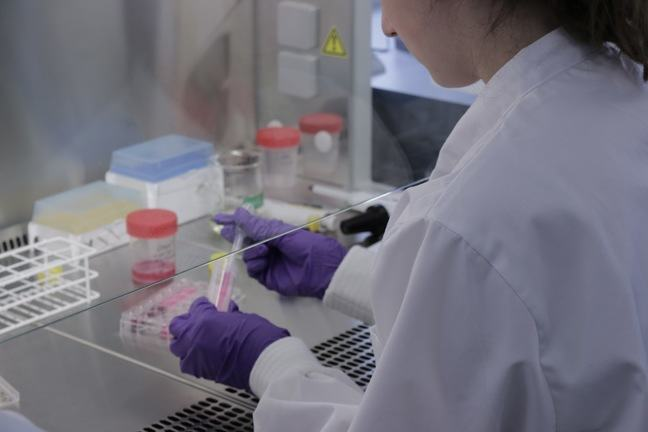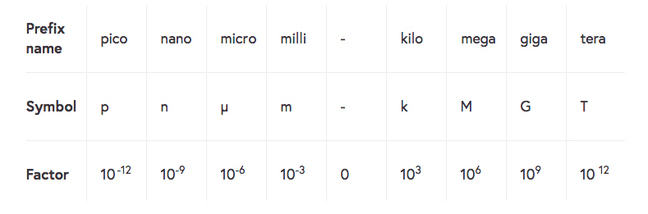## Want to keep learning?

This content is taken from the The University of Sheffield's online course, Technical Report Writing for Engineers. Join the course to learn more.
4.5

## The University of Sheffield# Units of measurement

Engineering is concerned with quantities, not numbers in isolation. As an engineer, you always consider quantities expressed as a number and a unit together. For example, a length is a quantity and can be expressed as a number of metres, where the unit is indicated by the word “metres”.

A length of about one metre can be defined as a multiple of many different units:

• 1.9 cubit
• 39.370 inch
• 52.5 unglie
• 1 650 763.73 wavelengths of the orange-red emission line in the electromagnetic spectrum of the krypton-86 atom in a vacuum

Although any one of these units may be correct, they may not always be understood by your reader. To ensure that quantities are understood throughout the world, an international set of units, SI (from the French: Système international d’unités), has been defined.

These are the most familiar units, for example metres (m), Volts (V) or Specific Entropy (J kg-1 K-1). There are seven base SI units and any number of derived ones. It’s vital that you use these whenever a value is reported.

Variables need to have their units defined at least once either in the nomenclature (as we saw in Week 2) or in the text where they are first used.

Whenever you report a value, you should include its units. There are two ways of writing units: using an exponent and using a slash sign. Both are acceptable, but you must be consistent throughout your report. This is particularly important if you are collaborating with others on a report.

### Here are some examples of SI Units:

Unit In words Using an exponent Using a slash (division sign)
Specific entropy Joules per kilogramme per Kelvin Jkg-1K-1 J/(kg K)
Acceleration Metres per second squared ms-2 m/s2
Viscosity Pascal seconds or Newton metres per second Pa.s or Nms-1 Pa.s or Nm/s

Table 1: Examples of some SI units.

Note that unit symbols remain unaltered in the plural, so there should be no confusion between pascal seconds, written as Pas, and the plural of pascals which is just Pa. However, it is a good idea to put a dot or space between the “Pa” and the “s” (Pa.s, or Pa s) to be clear.

Units can be modified by prefixing a letter which indicates that it is multiplied by a power of 10. For example, the amount of power produced from a large power station is 2 trillion (109) Joules per second, but we usually report this as 2GW.

### The most common prefixes are shown below:Table 2: Prefixes for SI units.

Note that the prefix indicating multiply by 1000 is a lowercase k. The uppercase K is the unit symbol for the kelvin, so 1 Kg denotes one kelvin gram, which is different to 1 kg, a kilogram.